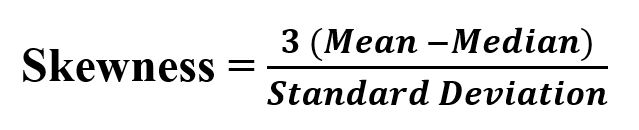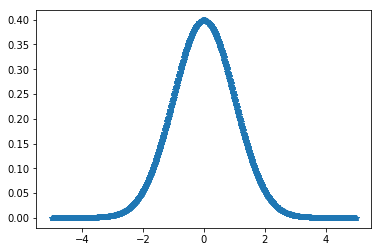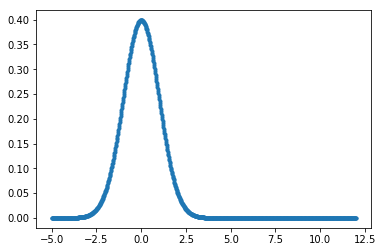Open In App

# scipy stats.skewtest() function | Python

`scipy.stats.skewtest(array, axis=0)` function test whether the skew is different from the normal distribution. This function tests the null hypothesis that the skewness of the population that the sample was drawn from is the same as that of a corresponding normal distribution.

Its formula –Parameters :
array : Input array or object having the elements.
axis : Axis along which the skewness test is to be computed. By default axis = 0.

Returns : Z-score (Statistics value) and P-value for the hypothesis test on data set.

Code #1:

 `# Performing skewtest``from` `scipy.stats ``import` `skewtest``import` `numpy as np ``import` `pylab as p `` ` `x1 ``=` `np.linspace( ``-``5``, ``5``, ``1000` `)``y1 ``=` `1.``/``(np.sqrt(``2.``*``np.pi)) ``*` `np.exp( ``-``.``5``*``(x1)``*``*``2`  `)`` ` `p.plot(x1, y1, ``'*'``)`` ` ` ` `print``( ``'\nSkewness test for given data :\n'``, skewtest(y1))`

Output :

```Skewness test for given data :
SkewtestResult(statistic=11.874007880556805, pvalue=1.6153913086650964e-32)
```

Code #2:

 `# Performing skewtest``from` `scipy.stats ``import` `skewtest``import` `numpy as np ``import` `pylab as p `` ` `x1 ``=` `np.linspace( ``-``5``, ``12``, ``1000` `)``y1 ``=` `1.``/``(np.sqrt(``2.``*``np.pi)) ``*` `np.exp( ``-``.``5``*``(x1)``*``*``2`  `)`` ` `p.plot(x1, y1, ``'.'``)`` ` ` ` `print``( ``'\nSkewness for data :\n'``, skewtest(y1))`

Output :

```Skewness for data :
SkewtestResult(statistic=16.957642860709516, pvalue=1.689888374767126e-64)
```